6.5 Concurrent lines of triangles:

Definition:

 The perpendicular drawn from the vertex of a triangle to its opposite side is called ‘altitude’.   In the adjoining figure C is the vertex. CM is perpendicular to the opposite side AB (extended). CM is called Altitude. Similarly we can draw 2 altitudes from other vertices (vertex A to the opposite side BC and vertex B to the opposite side AC). Note: Triangles have three altitudes.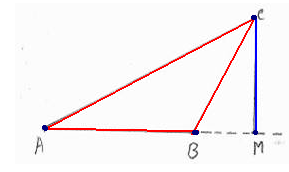Three or more straight lines are said to be ‘concurrent’ if they all pass through a common point. This common point is called ‘point of concurrency’.   In the adjoining figure, lines AB, CD, EF and GH pass through a common point (intersection) O. Hence all these four lines are concurrent and O is the point of concurrency.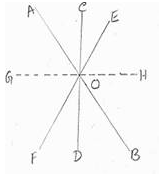Activity:  Construct few triangles and draw altitudes from each of the vertex of the triangle. Did you observe that altitudes are concurrent lines?

6.5.1 Construction of Altitudes:

 Step 1: Construct a triangle of given measurements (say AB =7.5cm, AC=4cm, BC =7cm)   Step 2: With vertex C as center, draw an arc of suitable radius to cut the opposite side AB at  2 points (if necessary extend the side) X, Y as shown in the figure.   Step 3: With X and Y as centers, draw arcs (of radius more than half of XY) to cut at point Z.   Step 4: Join C, Z to intersect the opposite side at L, CL is the altitude.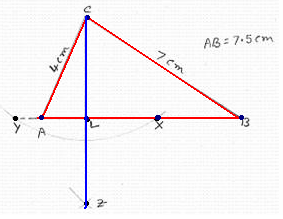Step 5:  With Vertex A as center draw an arc of suitable radius to cut the opposite side BC at 2 points (if necessary extend the side) H, G as shown in the figure.   Step 6: With H and G as centers draw arcs (of radius more than half of HG) to cut at point I.   Step 7: Join A, I to intersect the opposite side at N, AN is an altitude.   Step 8:  With Vertex B as center draw an arc of suitable radius to cut the opposite side AC  at 2 points (if necessary extend the side) E, D as shown in the figure.   Step 9: With E and D as centers draw arcs (of radius more than half of ED) to cut at point F.   Step 10: Join B, F to intersect the opposite side at M, BM is an altitude.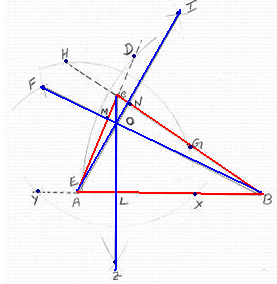We notice that all the three altitudes  intersect at a common point O. The point of concurrence of three perpendiculars drawn from the vertices of a triangle to their opposite sides is called ‘orthocenter’ and is usually denoted by ‘O’

Let us observe the position of orthocenter in three different types of triangles (obtuse, acute and Right angled triangle).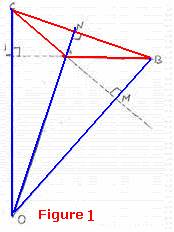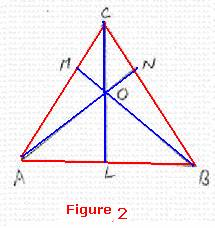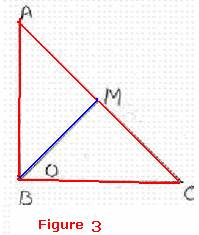In figure 1, ABC is an obtuse angled triangle and its orthocenter O, is outside the triangle. In figure 2, ABC is an acute angled triangle and its orthocenter O, is inside the triangle. In figure 3, ABC is a right angled triangle and its orthocenter O, is vertex of the right angle itself.

Definition:

 The line joining a vertex of the triangle to the middle point of the opposite side is called ‘median’. In the adjoining figure of triangle ABC A, B, C are the three vertices. L, M, N are midpoints of AB, BC and AC respectively. The line CL joining the vertex (C) to the mid point (L) of opposite side AB is called median. The line AM joining the vertex (A) to the mid point (M) of opposite side BC is called median. The line BN joining the vertex (B) to the mid point (N) of opposite side AC is called median.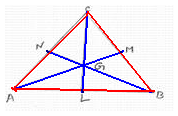6.5.2 Construction of Medians:

 Step 1: Construct a triangle of given measurements (say AB = 5cm, AC = 5cm and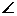CAB = 500).       Step 2: Bisect side AB (Draw arcs of radius more than half the length of AB on both sides of AB).   Let these arcs meet at X and Y.       Step 3: Join X and Y to cut the line AB at L. Join CL. CL is the median.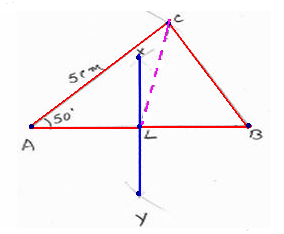Step 4: Bisect side BC (Draw arcs of radius more than half the length of BC on both sides of BC).   Let these arcs meet at P and Q.     Step 5: Join P and Q to cut the line BC at M. AM is the median     Similarly construct the median from Vertex B.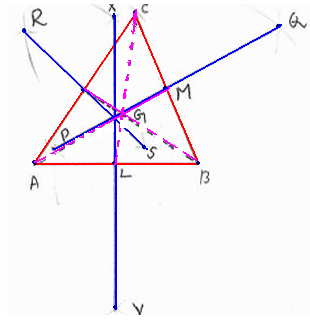Note: All the medians pass through a common point G. Definition: The point of concurrence of the three medians of a triangle is called ‘centroid’ and is usually denoted by ‘G’.

Let us look at the property of centroid for different types of triangles (Acute, Right and Obtuse angled triangles).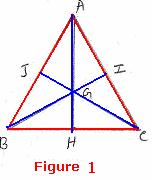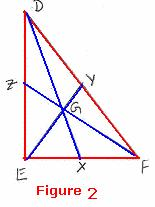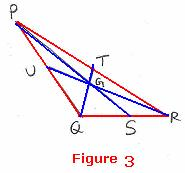Measure the distance of G from the vertex and center of opposite side. We notice the following: In  figure I (Acute angled triangle), 2GH = AG, 2GI= BG, 2GJ=GC. In  figure II (Right angled triangle), 2GX = DG, 2GY=EG, 2GZ=GF. In  Figure III (Obtuse angled triangle), 2GS=PG, 2GT=QG, 2UG=GR

Thus we conclude that the Centroid divides the median in the ratio of 2:1 with respect to the vertex and opposite side.

Definition: The bisector of any side of a triangle is called ‘perpendicular bisector’.

6.5.3 Construction of perpendicular bisector

 Step 1: Construct a triangle of given measurements (AB=7.5cm, ABC=450 ,AC=4cm)     Step2: With A,B as centers draw arcs of radius more than half the length of AB on both sides of AB. Let these arcs meet at X and Y.       Step3: Join XY to meet AB at L. (Note that XY bisects AB and is perpendicular to AB)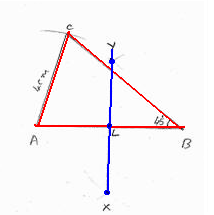Step4: With B,C as centers draw arcs of radius more than half the length of BC on both sides of BC.   Let these arcs meet at P and Q     Step 5: Join PQ to meet BC at M. (Note that PQ bisects BC and is perpendicular to BC)     Step6: With A,C as centers draw arcs of radius more than half the length of AC on both sides of AC.   Let these arcs meet at T and U.   Step 7: Join TU to meet AC at N. (Note that TU bisects AC and is perpendicular to AC)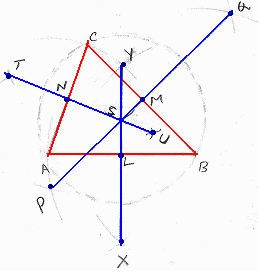Note that three perpendicular bisectors of a triangle are concurrent and they meet at S. Definition: The point of concurrence of the perpendicular bisectors of the sides of a triangle is called ‘Circmcenter’ and is normally denoted by ‘S’ or ‘C’.

We observe that any point on the perpendicular bisector XY is equidistance from both A and B, and hence the point S is equidistance from both A and B.

Similarly any point on the perpendicular bisector PQ is equidistance from both B and C, and hence the point S is equidistance from both B and C.

So we observe that SA=SB=SC.

If we draw a circle with S as center and SA as radius, we see that the this circle passes through all the vertices (A, B, C) of the triangle ABC.

Definition:

A circle which passes through all the vertices of a triangle is called ‘circumcircleof the triangle.

Let us look at the position of circumcentre in three different types of triangles (acute, right and obtuse angled triangle).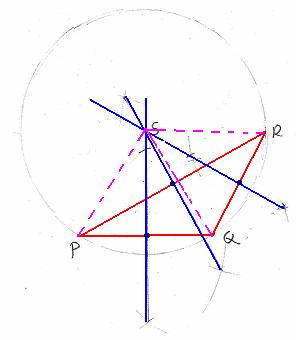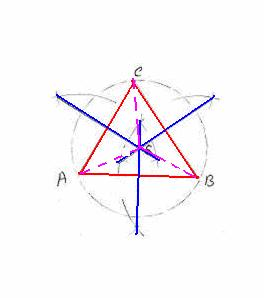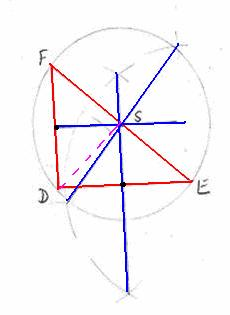Figure 1 Figure 2 Figure 3 In figure 1, PQR is an obtuse angled triangle and its circumcentre S is outside the triangle. In figure 21, ABC is an acute angled triangle and its circumcentre S is inside the triangle. In figure 32, DEF is a right angled triangle and its circumcentre S is on the hypotenuse

6.5.4 Construction of angular bisector:

 Step1: construct a triangle ABC with the given measurements.     Step2: With A as center, draw arcs of same radius to cut the sides AB and AC at P and Q respectively.     Step3: With P and Q as centers and with more than half of PQ as radius, draw arcs to intersect at R.     Step4: Join AR, this is the angular bisector ofCAB.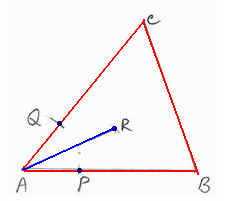Step5: With B as center, draw arcs of same radius to cut the sides BC and BA at T and S respectively   Step6: With T and S as centers and with more than half of TS as radius, draw arcs to intersect at U.   Step7: Join BU, this is the angular bisector ofABC.   Step8: With C as center, draw arcs of same radius to cut the sides CA and CB at W and V respectively.   Step9: With W and V as centers and with more than half of WV as radius, draw arcs to intersect at X.   Step10: Join CX, this is the angular bisector ofACB   Step 11: Mark the point of concurrence as I.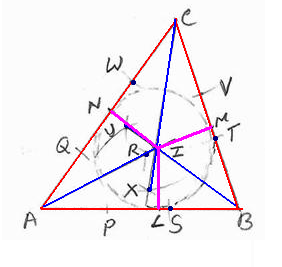Note that three angularperpendicular bisectors of a triangle are concurrent and they meet at I. Definition: The point of concurrence of three angular bisectors of a triangle is called ‘Incenter’ and is usually denoted by ‘I’.

6.5.5 Construction of Incircle:

 Step1: Construct incenter for the triangle as described above   Step 2: From I, draw perpendiculars to each of the 3 sides -AB, BC and CA and let they meet these sides at L,M and N respectively. Notice that IL=IM=IN.   Step 3: With I as center draw a circle of radius IL.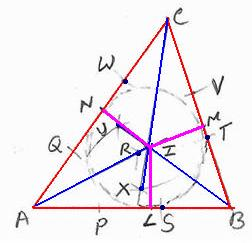Definition: The circle with Incenter of the triangle as the center and which touches the sides of the triangle is called ‘incircle’.

Note: In the case of an equilateral triangle,

Its orthocenter= it’s Incenter = it’s centroid= it’s Circumcenter (O=I=G=S)

 Concurrent Lines Point of concurrence Name Altitudes O Orthocenter Angular bisectors I Incenter Medians G Centroid(Center of gravity) Perpendicular bisectors S/C Sircumcenter

How to remember!!

OIGS stands for On India Government Services. It is for All (Altitudes) Indian (Angular) Middle(Medians) class People (Perpendicular bisectors).

Note: In section 6.13 we will be proving concurrency of these lines.

When we locate various points of concurrence in various types of triangles we observe:

 Point of concurrence Type of triangle Location of point of concurrence Centroid (G) Any type of triangle Inside the triangle In center(I) Any type of triangle Inside the triangle Circumcentre(C,S) Acute angled triangle Inside the triangle Right angled triangle Mid point of hypotenuse Obtuse angled triangle Outside the triangle Orthocenter(O) Acute angled triangle Inside the triangle Right angled triangle Vertex of right angle Obtuse angled triangle Outside the triangle

 As discussed above every triangle will have four concurrent points.   If that is the case. given a triangle shaped wooden object as shown in the adjacent figure, will it be possible to suspend the object in the air using a string passing through a point maintaining equilibrium status?   If possible which is that point?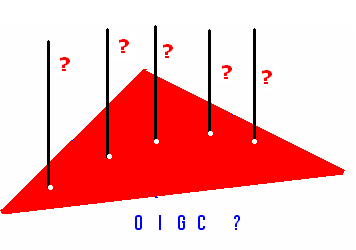6.5 Summary of learning

 No Points to remember 1 The perpendicular drawn from the vertex of a triangle to its opposite is called altitude. 2 Point of concurrency is a point through which, three or more concurrent lines pass through 3 Orthocenter(O) is the point of concurrency of the altitudes of a triangle 4 Centroid(G) is the point of concurrency of the medians of a triangle 5 Circmcenter(S) is the point of concurrency of the perpendicular bisectors of a triangle 6 Circumcircle is a circle which passes through all the vertices of a triangle and its center is Circmcenter(S) 7 In circle is the circle  which touches internally all the sides of a triangle and its center is  in center(I) which is point of concurrence of angular bisectors.